Thermos Index

 Introduction The steady flow energy equation relates to open systems working under steady conditions i.e in which conditions do not change with time. The boundary encloses a system through which fluid flows at a constant rate, whilst heat transfer occurs and external work is done all under steady conditions ,that is , the rates of mass flow and energy flow are constant with respect to time. The equation for steady flow ( the steady flow energy equation ) is generally written per unit mass as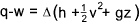q = heat transfer across boundary per unit mass w = external work done by system per unit mass z = fluid height v = fluid velocity h = fluid enthalpy ( u (internal energy + pv (pressure.specific volume) Note in the examples below the system control volumes are defined by the red dashed line. Heater.. The steady flow equation as applied to a fluid heater.. Potential energy (z) assumed to be constant.. Kinetic energy changes (1 to 2) assumed to be very small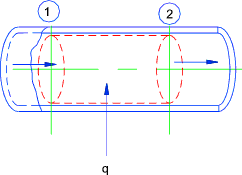Heater w = 0 therefore q = h2-h1 Turbine ..(Assumed Adiabetic Expansion)..The steady flow equation as applied to a turbine.. Potential energy (z) assumed to be constant.. Kinetic energy changes (1 to 2) assumed to be very small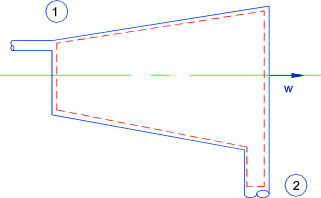Turbine q = 0 therefore w = h2-h1 Throttling ..(Assumed Adiabetic )...The steady flow equation as applied to a orifice.. Potential energy (z) assumed to be constant.. The higher velocity at orifice section is dissipated in tube downstream of the orifice and therefore the kinetic energies at 1 and 2 are similar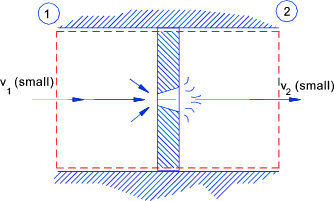Orifice q = w = 0 therefore therefore h1 = h2 Nozzle..(Assumed Adiabetic )...The steady flow equation as applied to smooth nozzle.. Potential energy (z) assumed to be constant.. Kinetic energy changes are assumed to be significant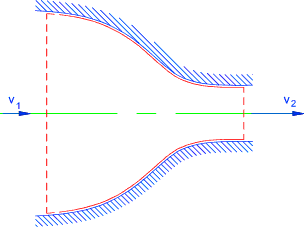Nozzle q = w = 0 therefore (v22 - v12 ) /2 = (h1 - h2 )